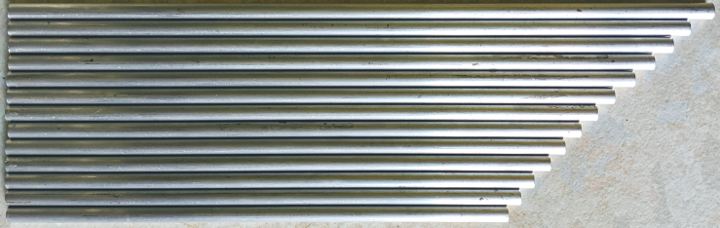## 20200422

### The results are in

As promised in the last post, we have now calculated the lengths of aluminium tubing (of a certain grade) required to produce a set of tubular chimes - and even that is much too grandiose a term for the rather toy-like set of factory-cut hollow rods purchased, at the very reasonable price of about thirty quid (including delivery), for the experiment.

The material arrived the next day, and I measured the lengths principally because I needed accurate data for my calculations below - and only secondarily to check that they were as ordered (which they pretty much were, and certainly within the vendor's advertised tolerance). I also weighed them, principally to check that the density matched the theoretical density used in the frequency calculations - which of course it did, to within about a half of a percent. As I have no machinery to check its Young's modulus of elasticity, I continue to rely on its wikipedially reported value.

Measuring their frequencies was the most difficult task, and involved suspending the tubes with a hot-glued thread connecting the top of each tube to a shelf, and recording them - with my trusty H2N Zoom recorder - being lightly struck by a similar aluminium tube. Here's a composite of the noises made by this 'instrument'.

Do not be fooled by the levels. Although the samples in this recording are untreated (apart from being chopped up into segments), those small cylinders cannot shift very much air and the sounds are quite quiet. It's the H2N being held (by hand) up close.

The samples were brought into Cockos Reaper whereupon the use of its built-in band-pass filter, ReaEQ, (to cut out the highly audible harmonics) and frequency counter (ReaTune) plugins proved adequate to the task. Here are all the measurements, plus a picture of the set of tubes.

lengthweightfrequency$\kappa$13 Pipelings
($mm$)($gm$)(Hz)($m^2\,s^{-1}$)(png)
25110.6351432.424210.2355132.3
2369.9657932.2
2299.7562032.5
2229.4365132.1
2159.1569832.3
2108.8173432.4
2038.678632.4
1988.3282132.2
1938.1486632.3
1877.8792032.2
1827.6997032.1
1777.51101831.9

As expected, the frequencies were not even close to the design targets (respectively 165, 175, 185, 196, 208, 220, 233, 247, 262, 277, 294, 311, 330) and were - on average - 3.14 times higher (to the three significant figures employed throughout this exercise). The final column in the above table is $\kappa$, the diffusivity mentioned in the previous post, and is calculated as $L^2 \times f$ for each row ($L$ being the metre value). The average value for $\kappa$ is 32.2, the same multiple (3.14, weirdly close to $\pi$) of the value of 10.2 expected.

But - and also as expected - the instrument does indeed play a very serviceable chromatic scale. It may not start on the 165 Hertz concert pitch E (as requested) but instead a rather higher 514 Hertz non-concert pitch flat C, not quite a quarter tone below the 'proper' one. That's quite a way out, but our measured value of $\kappa$ could now be used to calculate a new series of $L$ values from $L=\sqrt{\kappa/f}$ - provided of course we were to use exactly the same material. All lengths would, accordingly, be multiplied by a factor of 1.77 (the square root of 3.14).

For this particular kind of aluminium alloy the previous post shows that $\kappa = 1420 \sqrt{D_o^2 + D_i^2}$, where $D_o = 0.006 m$ (i.e. 6mm) and $D_i = 0.004 m$ (i.e. 4mm). It's perfectly reasonable to incorporate the 'blame' for the (fudge) factor of 3.14 into the 'material constant' of 1420 and claim that in practice this constant should be 4450. This leaves us free to use larger inner and outer diameters as long as it's the same alloy to allow us to shift more air and make louder instruments. For example, if we have our heart set on a more robust 165 Hertz concert pitch E, we choose a larger diameter tubing (say half an inch with a 6.2mm bore). This would give us a new value for $\kappa$ - based on $D_o = 0.0127\,m$ and $D_i=0.0062\,m$ - of $4450 \times 0.01413 = 63$. From this we'd calculate a length of 618 mm.

And so on

### Fudge

So why was the calculation incorrect? Why would we need to use 4450 rather than 1420? As the 1420 was itself calculated - as $(22.4/8\pi) \sqrt{E/\rho}$ - from easily verifiable physical properties of a particular alloy of aluminium, the only places where the discrepancy could occur are in the value of 22.4 and from the way that $I$ was defined (in terms of $D_o$ and $D_i$). Perhaps an engineer can let me know where I went wrong?

Finally, here's a plot of frequency versus length of the tubes received in the mail. The least squares fit (from LibreCalc) is $f = 33.6 \times L^{-1.97}$, satisfyingly close to the model of $L^2 f = \kappa$ (with my measured average of $\kappa=32.2$).